Fubini theorem

(diff) ← Older revision | Latest revision (diff) | Newer revision → (diff)

A theorem that establishes a connection between a multiple integral and a repeated one. Suppose that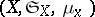and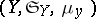are measure spaces with-finite complete measures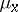anddefined on the-algebrasand, respectively. If the functionis integrable on the product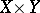ofandwith respect to the product measure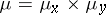ofand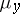, then for almost-all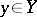the functionof the variableis integrable onwith respect to, the function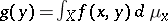is integrable onwith respect to, and one has the equality(1)

Fubini's theorem is valid, in particular, for the case when,andare the Lebesgue measures in the Euclidean spaces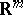,andrespectively (andare natural numbers),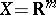,,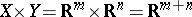, and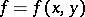is a Lebesgue-measurable function on,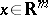,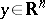. Under these assumptions, formula (1) has the form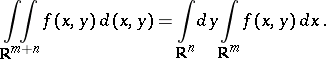(2)

In the case of a functiondefined on an arbitrary Lebesgue-measurable set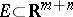, in order to express the multiple integral in terms of a repeated one, one must extendby zero to the whole of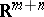and apply (2). See also Repeated integral.

The theorem was established by G. Fubini .

How to Cite This Entry:
Fubini theorem. Encyclopedia of Mathematics. URL: http://encyclopediaofmath.org/index.php?title=Fubini_theorem&oldid=17242
This article was adapted from an original article by L.D. Kudryavtsev (originator), which appeared in Encyclopedia of Mathematics - ISBN 1402006098. See original article